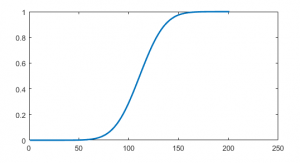11
Mar 17

## Distribution function propertiesThe word "distribution" is repeated in elementary Stats texts hundreds of times yet the notion of a distribution function is usually mentioned tangentially or not studied at all. In fact, the distribution function is as important as the density and in binary choice models it is the king. The full name is a cumulative distribution function (cdf) but I am going to stick to the short name (used in advanced texts). This is one of the topics most students don't get on the first attempt (I was not an exception).

### Motivating example

Example. Electricity consumption sharply increases when everybody starts using air conditioners, and this happens when temperature exceeds$20\,^{\circ}C$. The utility company would like to know the likelihood of a jump in electricity consumption tomorrow at noon.

1. Consider the probability$P(T \le 15)$ that the temperature tomorrow at noon$T$ will not exceed$15\,^{\circ}C$. How does it relate to the probability$P(T \le 20)$? The second probability is obviously larger, and this can be visualized by comparing the intervals$(-\infty,15]$ and$(-\infty,20]$.
2. Suppose in the expression$P(T \le t)$ the real number$t$ increases to$+\infty$. What happens to the probability? As the intervals extend to the right, they eventually include all possible temperatures, and the probability$P(T \le t)$ approaches 1.
3. Now think about$t$ going to$-\infty$. Then what happens to$P(T \le t)$? It's the opposite of the previous case. Eventually, all possible temperatures are excluded, and the probability$P(T \le t)$ goes to 0.

### Generalization

Definition. Let$X$ be a random variable and$x$ a real number. The distribution function$F_X$ of$X$ is defined by$F_X(x)=P(X\le x)$ (the random variable$X$ is fixed and therefore put in the subscript, whereas the real number$x$ changes and serves as the argument).

### Distribution function properties

1.$F_X(x)$ is the probability of the event$\{ X\le x\}$, so the value$F_X(x)$ always belongs to [0,1].
2. As the event becomes wider, the probability increases. This property is called monotonicity and is formally written as follows: if$x_1\le x_2$, then$\{X\le x_1\}\subset\{X\le x_2\}$ and$F_X(x_1)\le F_X(x_2)$.
3. As$x$ goes to$+\infty$, the event$\{X\le x\}$ approaches a sure event$\{X<+\infty\}=R$ and$F_X(x)$ tends to 1.
4. As$x$ goes to$-\infty$, the event$\{X\le x\}$ approaches an impossible event$\{X=-\infty\}=\varnothing$ and$F_X(x)$ tends to 0.Figure 1. Distribution function of a normal variable

From this we conclude that the graph of the distribution function may look as in Figure 1.

### Interval formula in terms of the distribution function

In many applications we are interested in probability of an event that$X$ takes values in an interval$\{a. Such probability can be expressed in terms of the distribution function. Just apply the additivity rule to the set equation$\{-\infty to get$F_X(b)=F_X(a)+P(a and, finally,

(1)$P(a

Definition. Equation (1) can be called an interval formula.